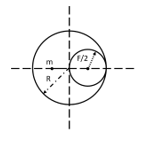Gravitational field intensity
Question

# A cavity of radius R/2 is made inside a solid sphere of radius R. The centre of the cavity is located at a distance R/2 from the centre of the sphere. The gravitational force on a particle of mass ‘m’ at a distance R/2 from the centre of the sphere on the line joining both the centres of sphere and cavity is (opposite to the centre of cavity) [Here $\mathrm{g}=\mathrm{GM}/{\mathrm{R}}^{2}$ , where M is the mass of the sphere]

Difficult
Solution

##${\mathrm{g}}_{\mathrm{net}}={\mathrm{g}}_{1}-{\mathrm{g}}_{2}$$\begin{array}{l}=\frac{\mathrm{Gm}\mathrm{R}}{2}}{{\mathrm{R}}^{3}}-\frac{\mathrm{G}\left(\mathrm{m}}{8}\right)}{{\mathrm{R}}^{2}}\\ =\frac{\mathrm{Gm}}{2{\mathrm{R}}^{2}}-\frac{\mathrm{Gm}}{8{\mathrm{R}}^{2}}\\ =\frac{\mathrm{Gm}}{2{\mathrm{R}}^{2}}\left[1-\frac{1}{4}\right]\\ =\frac{\mathrm{Gm}}{2{\mathrm{R}}^{2}}\left[\frac{3}{4}\right]\\ =\frac{3\mathrm{Gm}}{8{\mathrm{R}}^{2}}\end{array}$$\begin{array}{l}\mathrm{F}=\mathrm{mg}\\ =\frac{3\mathrm{GMm}}{8{\mathrm{R}}^{2}}=\frac{3\mathrm{mg}}{8}\end{array}$

Get Instant Solutions